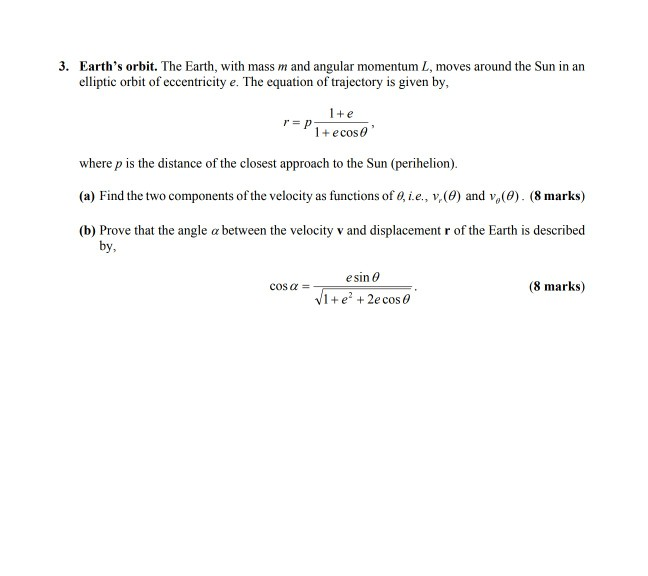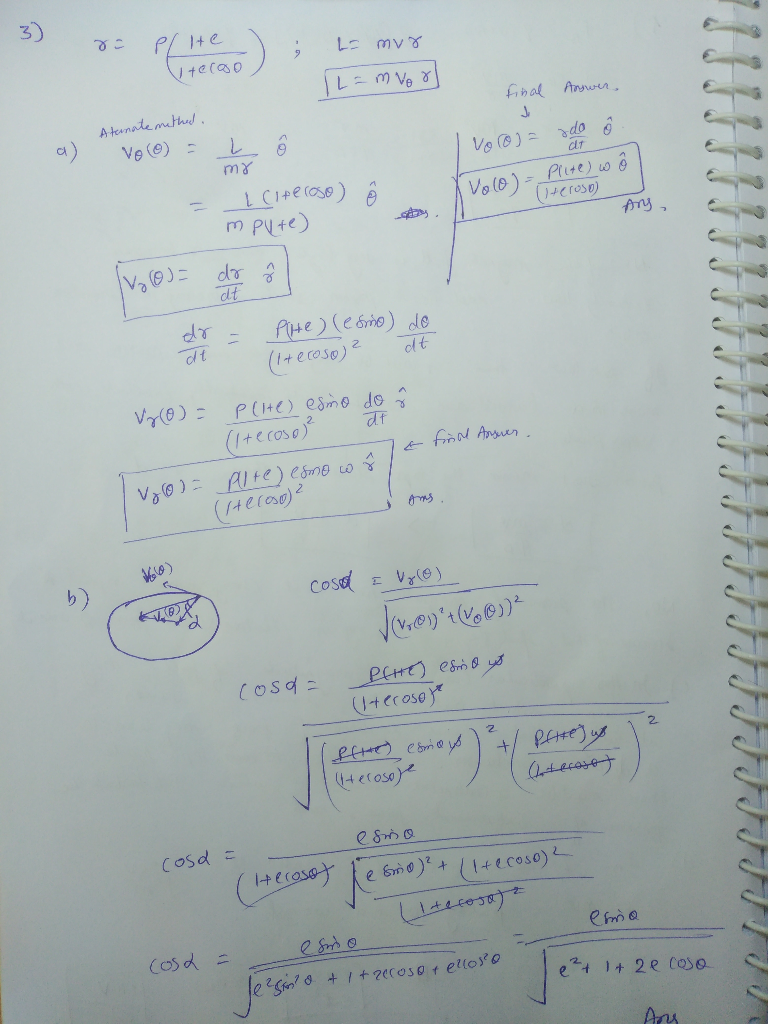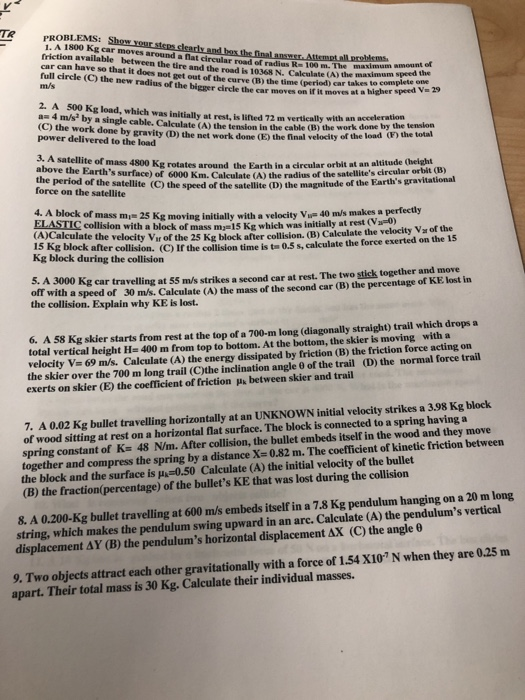Question

# 3. Earth's orbit. The Earth, with mass m and angular momentum L, moves around the Sun in arn elli...Accidently uploaded the photo in a recent question and need help solving this one.

3. Earth's orbit. The Earth, with mass m and angular momentum L, moves around the Sun in arn elliptic orbit of eccentricity e. The equation of trajectory is given by, r=p1+ecoso 1+e where p is the distance of the closest approach to the Sun (perihelion) (a) Find the two components of the velocity as functions ofQie, v,(9) and vo(9). (8 marks) (b) Prove that the angle α between the velocity v and displacement r of the Earth is described by, esin 1+e2 + 2e cos θ cos α = (8 marks)#### Earn Coins

Coins can be redeemed for fabulous gifts.

Similar Homework Help Questions
• ### The semimajor axis of Mars orbit is about 1.52 astronomical units (au), where an au is the Earth's average distance from the Sun, meaning the semimajor axis of Earth's orbit is 1 au. To go from Earth to Mars and use the least energy from rocket fuel, th

The semimajor axis of Mars orbit is about 1.52 astronomical units (au), where an au is the  Earth's average distance from the Sun, meaning the semimajor axis of Earth's orbit is 1 au.  To go from Earth to Mars and use the least energy from rocket fuel, the orbit has a semimajor axis of 1.26 au and an eccentricity of about 0.21.  Starting at Earth's orbit, to follow this path we give the spacecraft an orbital velocity of 40 km/s. ...

• ### An earth satellite moves in a circular orbit with an orbital speed of 5800 m/s

An earth satellite moves in a circular orbit with an orbital speed of 5800 m/s. Find the time (expressed in seconds) of one revolution of the satellite. Find the radial acceleration of the satellite in its orbit.I think we need to know the radius of the earth and the satellite's average distance from the earth in order to answer the question. All we have right now is the angular velocity. If you have that information, then use d=v*t where d...

• ### TR PROBLEMS: Show your 1. A 1800 Kg car moves around a flat circular road of...TR PROBLEMS: Show your 1. A 1800 Kg car moves around a flat circular road of radius R- 100 m friction available between the tire and the road is 10368 N. Calculate (A) car can have so that it does not get out of the curve (B) the time alLoroblems radius R-100 m. The maximum amount ef (C) the new radius of the bigger circle the car moves on if it moves at a higher s the maxinmum speed the...